International Tables for Crystallography (2006). Vol. B. ch. 2.4, pp. 264-275   | 1 | 2 | https://doi.org/10.1107/97809553602060000557

## Contents

• 2.4. Isomorphous replacement and anomalous scattering  (pp. 264-275)
• 2.4.1. Introduction  (p. 264) | html | pdf |
• 2.4.2. Isomorphous replacement method  (pp. 264-265) | html | pdf |
• 2.4.2.1. Isomorphous replacement and isomorphous addition  (pp. 264-265) | html | pdf |
• 2.4.2.2. Single isomorphous replacement method  (p. 265) | html | pdf |
• 2.4.2.3. Multiple isomorphous replacement method  (p. 265) | html | pdf |
• 2.4.3. Anomalous-scattering method  (pp. 265-269) | html | pdf |
• 2.4.3.1. Dispersion correction  (pp. 265-266) | html | pdf |
• 2.4.3.2. Violation of Friedel's law  (pp. 266-267) | html | pdf |
• 2.4.3.3. Friedel and Bijvoet pairs  (p. 267) | html | pdf |
• 2.4.3.4. Determination of absolute configuration  (pp. 267-268) | html | pdf |
• 2.4.3.5. Determination of phase angles  (p. 268) | html | pdf |
• 2.4.3.6. Anomalous scattering without phase change  (p. 268) | html | pdf |
• 2.4.3.7. Treatment of anomalous scattering in structure refinement  (pp. 268-269) | html | pdf |
• 2.4.4. Isomorphous replacement and anomalous scattering in protein crystallography  (pp. 269-274) | html | pdf |
• 2.4.4.1. Protein heavy-atom derivatives  (p. 269) | html | pdf |
• 2.4.4.2. Determination of heavy-atom parameters  (pp. 269-270) | html | pdf |
• 2.4.4.3. Refinement of heavy-atom parameters  (pp. 270-271) | html | pdf |
• 2.4.4.4. Treatment of errors in phase evaluation: Blow and Crick formulation  (pp. 271-272) | html | pdf |
• 2.4.4.5. Use of anomalous scattering in phase evaluation  (pp. 272-273) | html | pdf |
• 2.4.4.6. Estimation of r.m.s. error  (pp. 273-274) | html | pdf |
• 2.4.4.7. Suggested modifications to Blow and Crick formulation and the inclusion of phase information from other sources  (p. 274) | html | pdf |
• 2.4.4.8. Fourier representation of anomalous scatterers  (p. 274) | html | pdf |
• 2.4.5. Anomalous scattering of neutrons and synchrotron radiation. The multiwavelength method  (pp. 274-275) | html | pdf |
• 2.4.5.1. Neutron anomalous scattering  (p. 275) | html | pdf |
• 2.4.5.2. Anomalous scattering of synchrotron radiation  (p. 275) | html | pdf |
• References | html | pdf |
• Figures
• Fig. 2.4.2.1. Vector relationship betweenand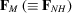(p. 265) | html | pdf |
• Fig. 2.4.2.2. Relationship between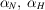and(p. 265) | html | pdf |
• Fig. 2.4.2.3. Harker construction when two heavy-atom derivatives are available  (p. 266) | html | pdf |
• Fig. 2.4.3.1. Variation of ( a )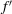and ( b )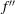as a function of atomic number for Cuand Moradiations  (p. 266) | html | pdf |
• Fig. 2.4.3.2. Vector diagram illustrating the violation of Friedel's law when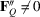(p. 267) | html | pdf |
• Fig. 2.4.3.3. A composite view of the vector relationship between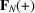and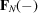(p. 267) | html | pdf |
• Fig. 2.4.4.1. Distribution of intersections in the Harker construction under non-ideal conditions  (p. 272) | html | pdf |
• Fig. 2.4.4.2. Vector diagram indicating the calculated structure factor,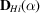, of the i th heavy-atom derivative  (p. 272) | html | pdf |
• Fig. 2.4.4.3. The probability distribution of the protein phase angle  (p. 272) | html | pdf |
• Fig. 2.4.4.4. Harker construction using anomalous-scattering data from a single derivative  (p. 273) | html | pdf |
• Tables
• Table 2.4.3.1. Phase angles of different components of the structure factor in space group(p. 267) | html | pdf |